#【机器学习PAI实战】—— 玩转人工智能之利用GAN自动生成二次元头像

1
6
21. 云栖社区>
2. 博客>
3. 正文

## 前言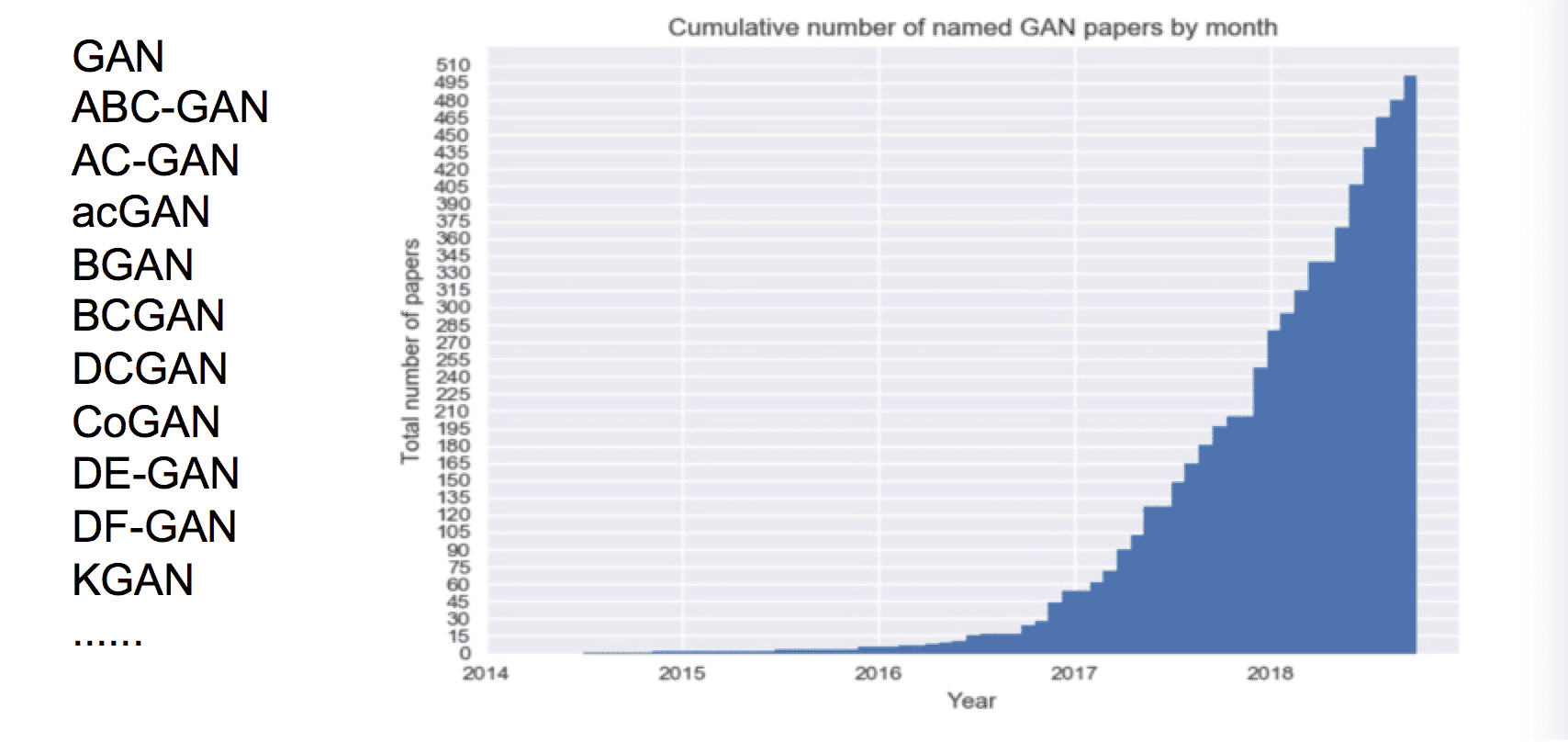## GAN简介

GAN主要的应用是自动生成一些东西，包括图像和文本等，比如随机给一个向量作为输入，通过GAN的Generator生成一张图片，或者生成一串语句。Conditional GAN的应用更多一些，比如数据集是一段文字和图像的数据对，通过训练，GAN可以通过给定一段文字生成对应的图像。

GAN主要可以分为Generator（生成器）和Discriminator（判别器）两个部分，其中Generator其实就是一个神经网络，输入一个向量，可以输出一张图像（即一个高维的向量表示），如下图示。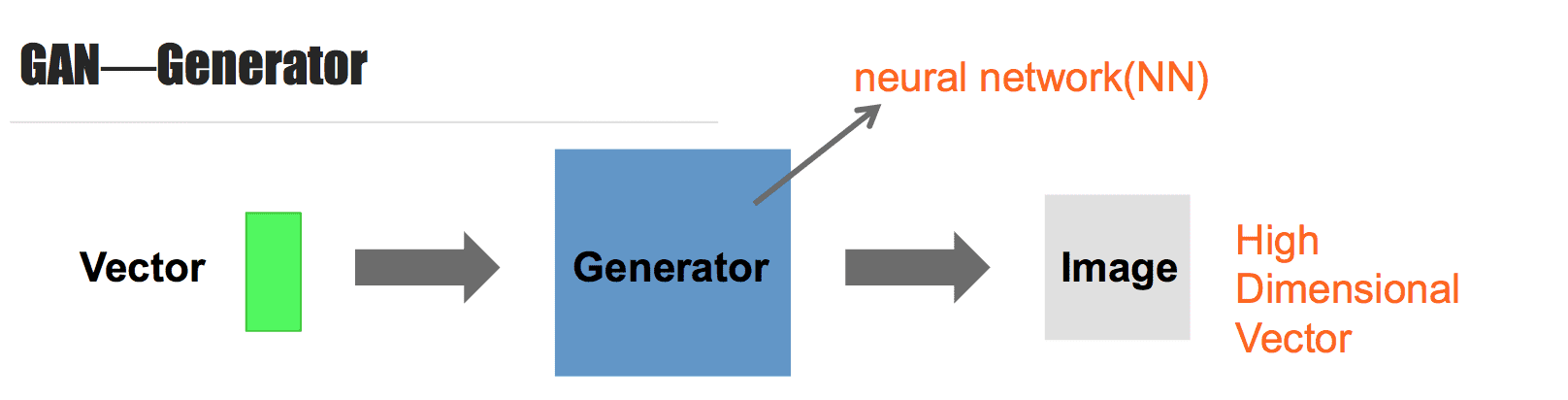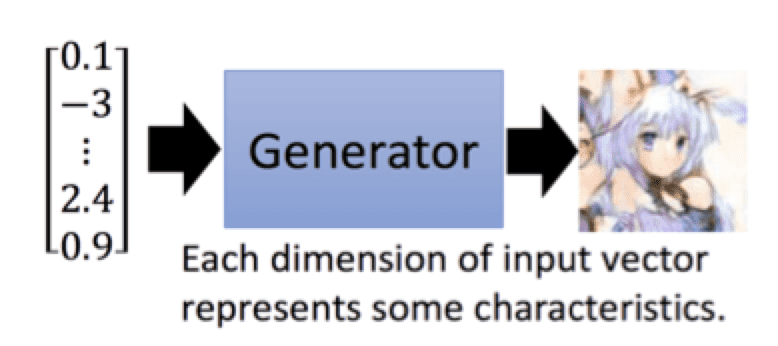Discriminator也是一个神经网络，输入为一张图像，输出为一个数值，输出的数值用于判断输入的图像是否是真的，数值越大，说明图像是真的，数值越小，说明图像为假的，如下图示。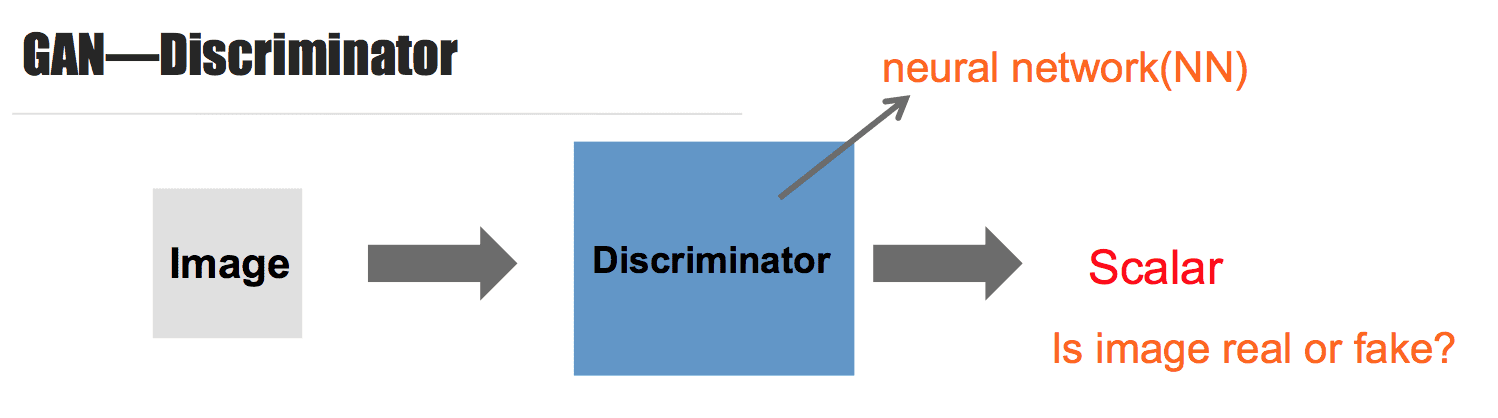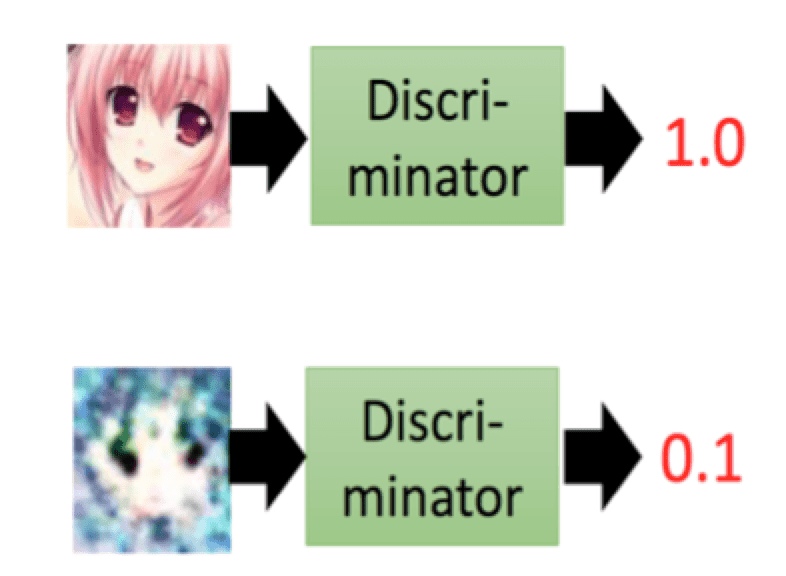Generator负责生成图像，Discriminator负责对Generator生成的图像和真实图像去进行对比，区别出真假，Generator需要不断优化来欺骗Discriminator，以假乱真；而Discriminator也不断优化，来提高识别能力，能够识别出Generator的把戏。二者的这种关系可以形象地通过下图展示。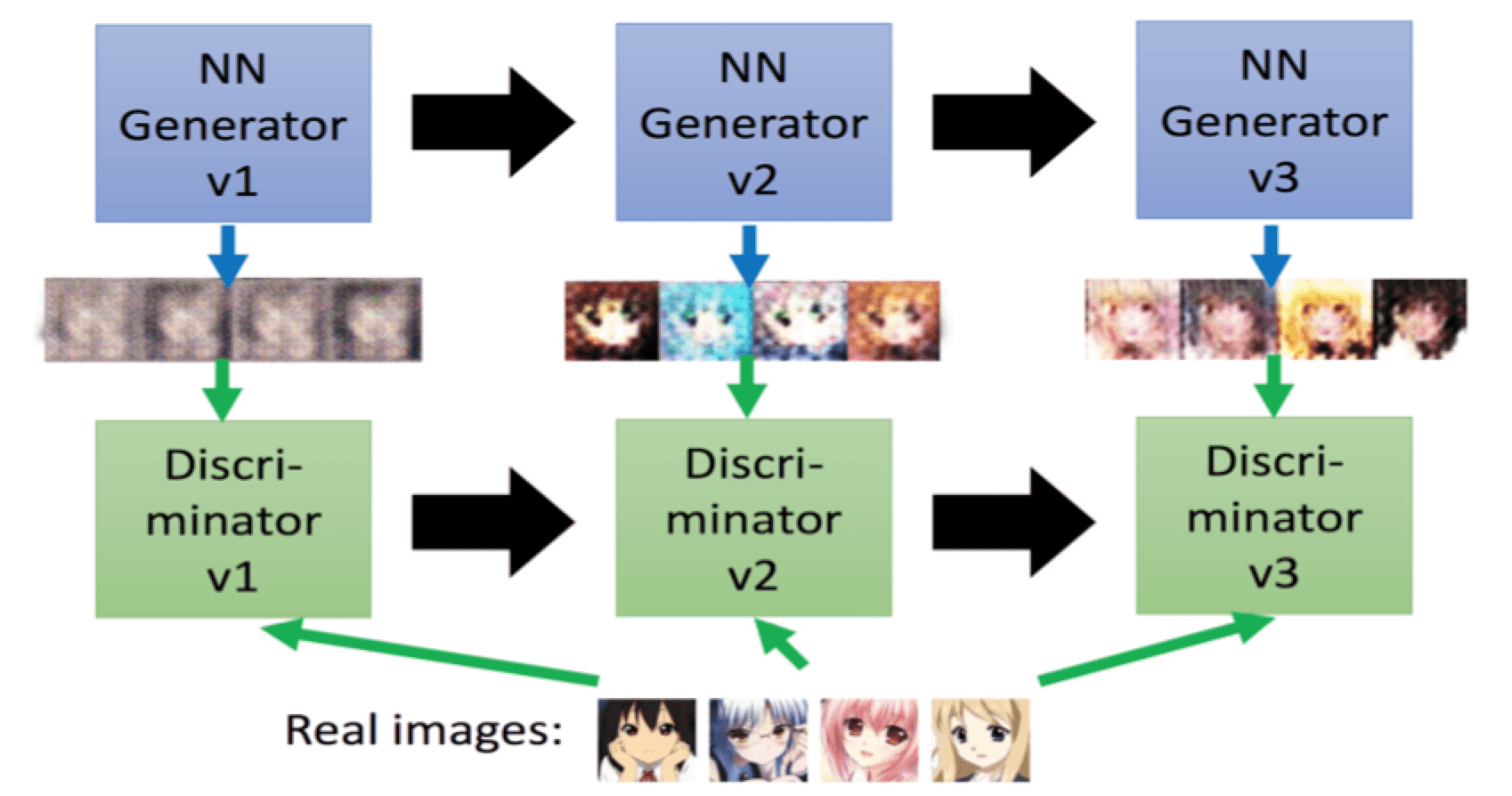Generator和Discriminator连接起来，形成一个比较大的深层网络，即为GAN网络。

## 数据准备## 算法实践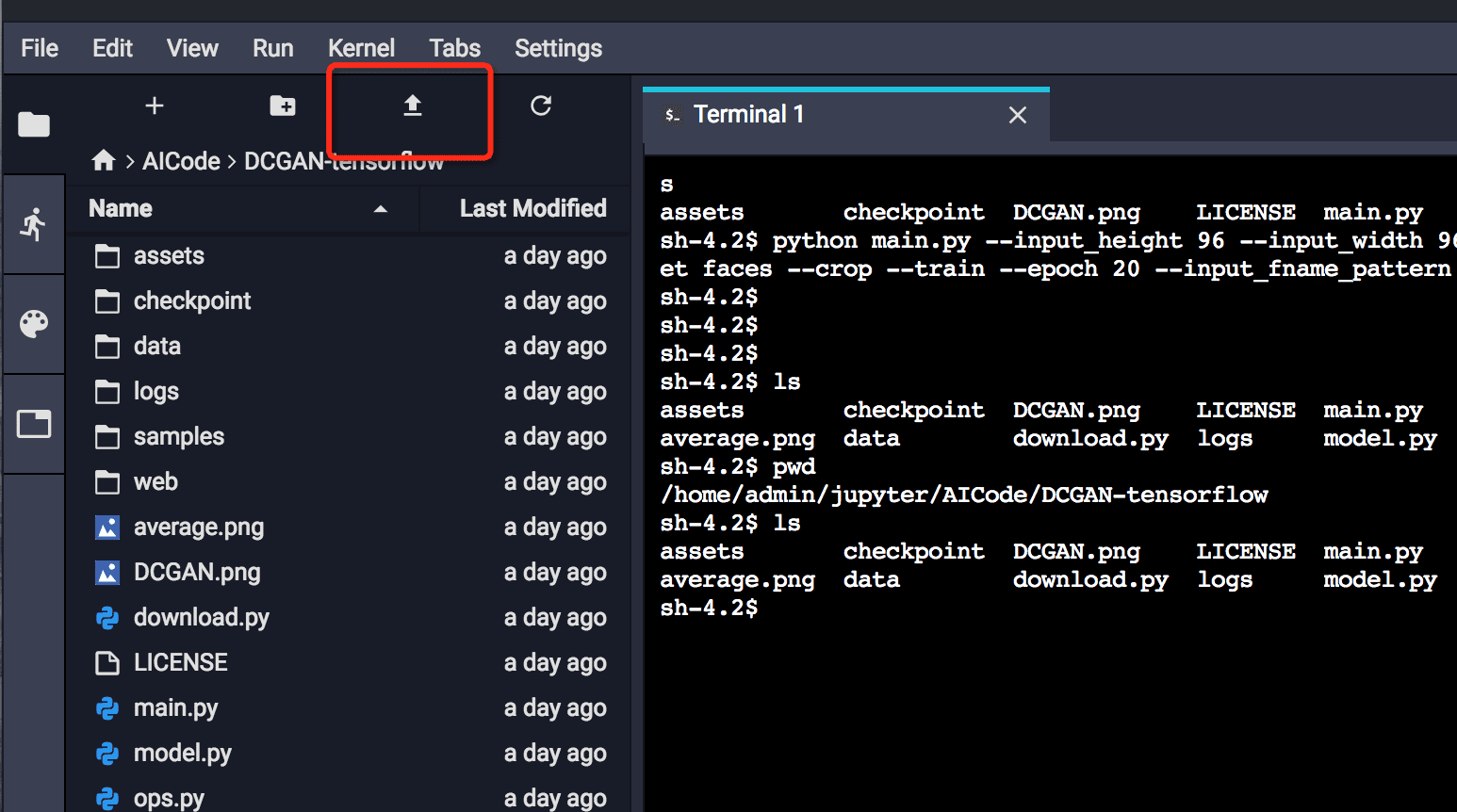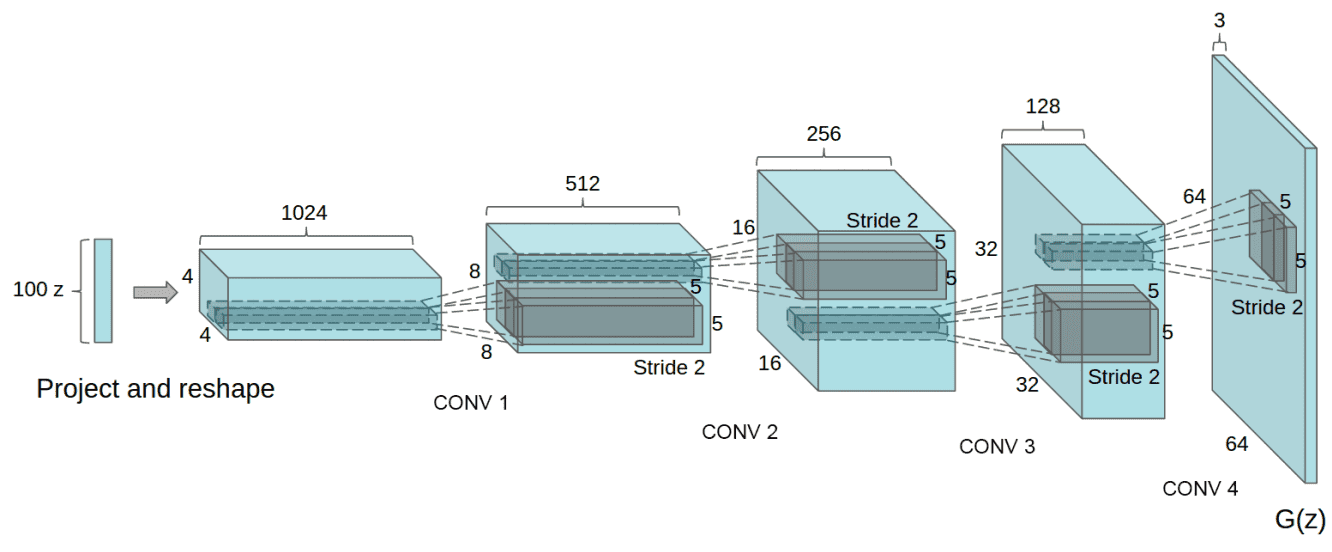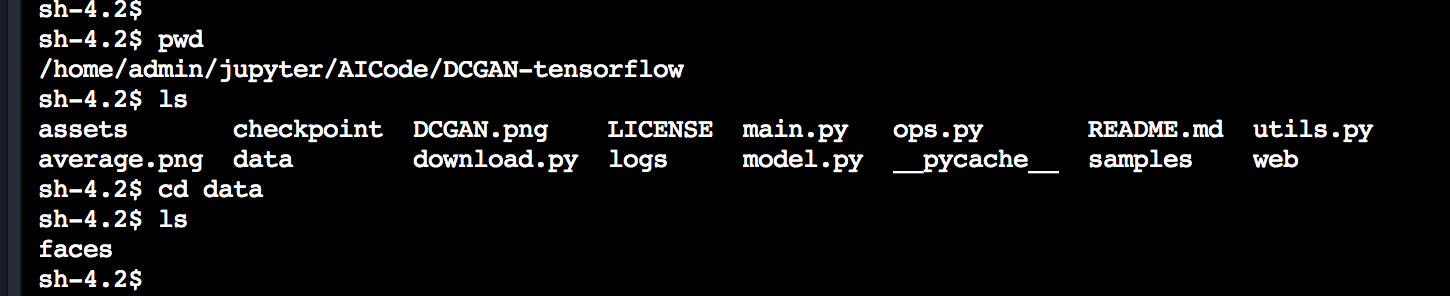``````def main(_):
pp.pprint(flags.FLAGS.__flags)

if FLAGS.input_width is None:
FLAGS.input_width = FLAGS.input_height
if FLAGS.output_width is None:
FLAGS.output_width = FLAGS.output_height

if not os.path.exists(FLAGS.checkpoint_dir):
os.makedirs(FLAGS.checkpoint_dir)
if not os.path.exists(FLAGS.sample_dir):
os.makedirs(FLAGS.sample_dir)

#gpu_options = tf.GPUOptions(per_process_gpu_memory_fraction=0.333)
run_config = tf.ConfigProto()
run_config.gpu_options.allow_growth=True

with tf.Session(config=run_config) as sess:
if FLAGS.dataset == 'mnist':
dcgan = DCGAN(
sess,
input_width=FLAGS.input_width,
input_height=FLAGS.input_height,
output_width=FLAGS.output_width,
output_height=FLAGS.output_height,
batch_size=FLAGS.batch_size,
sample_num=FLAGS.batch_size,
y_dim=10,
z_dim=FLAGS.generate_test_images,
dataset_name=FLAGS.dataset,
input_fname_pattern=FLAGS.input_fname_pattern,
crop=FLAGS.crop,
checkpoint_dir=FLAGS.checkpoint_dir,
sample_dir=FLAGS.sample_dir,
data_dir=FLAGS.data_dir)
else:
dcgan = DCGAN(
sess,
input_width=FLAGS.input_width,
input_height=FLAGS.input_height,
output_width=FLAGS.output_width,
output_height=FLAGS.output_height,
batch_size=FLAGS.batch_size,
sample_num=FLAGS.batch_size,
z_dim=FLAGS.generate_test_images,
dataset_name=FLAGS.dataset,
input_fname_pattern=FLAGS.input_fname_pattern,
crop=FLAGS.crop,
checkpoint_dir=FLAGS.checkpoint_dir,
sample_dir=FLAGS.sample_dir,
data_dir=FLAGS.data_dir)

show_all_variables()

if FLAGS.train:
dcgan.train(FLAGS)``````

``````        else:
# Update D network
_, summary_str = self.sess.run([d_optim, self.d_sum],
feed_dict={ self.inputs: batch_images, self.z: batch_z })

# Update G network
_, summary_str = self.sess.run([g_optim, self.g_sum],
feed_dict={ self.z: batch_z })

# Run g_optim twice to make sure that d_loss does not go to zero (different from paper)
_, summary_str = self.sess.run([g_optim, self.g_sum],
feed_dict={ self.z: batch_z })

errD_fake = self.d_loss_fake.eval({ self.z: batch_z })
errD_real = self.d_loss_real.eval({ self.inputs: batch_images })
errG = self.g_loss.eval({self.z: batch_z})``````

python main.py --input_height 96 --input_width 96 --output_height 48 --output_width 48 --dataset faces --crop --train --epoch 300 --input_fname_pattern "*.jpg"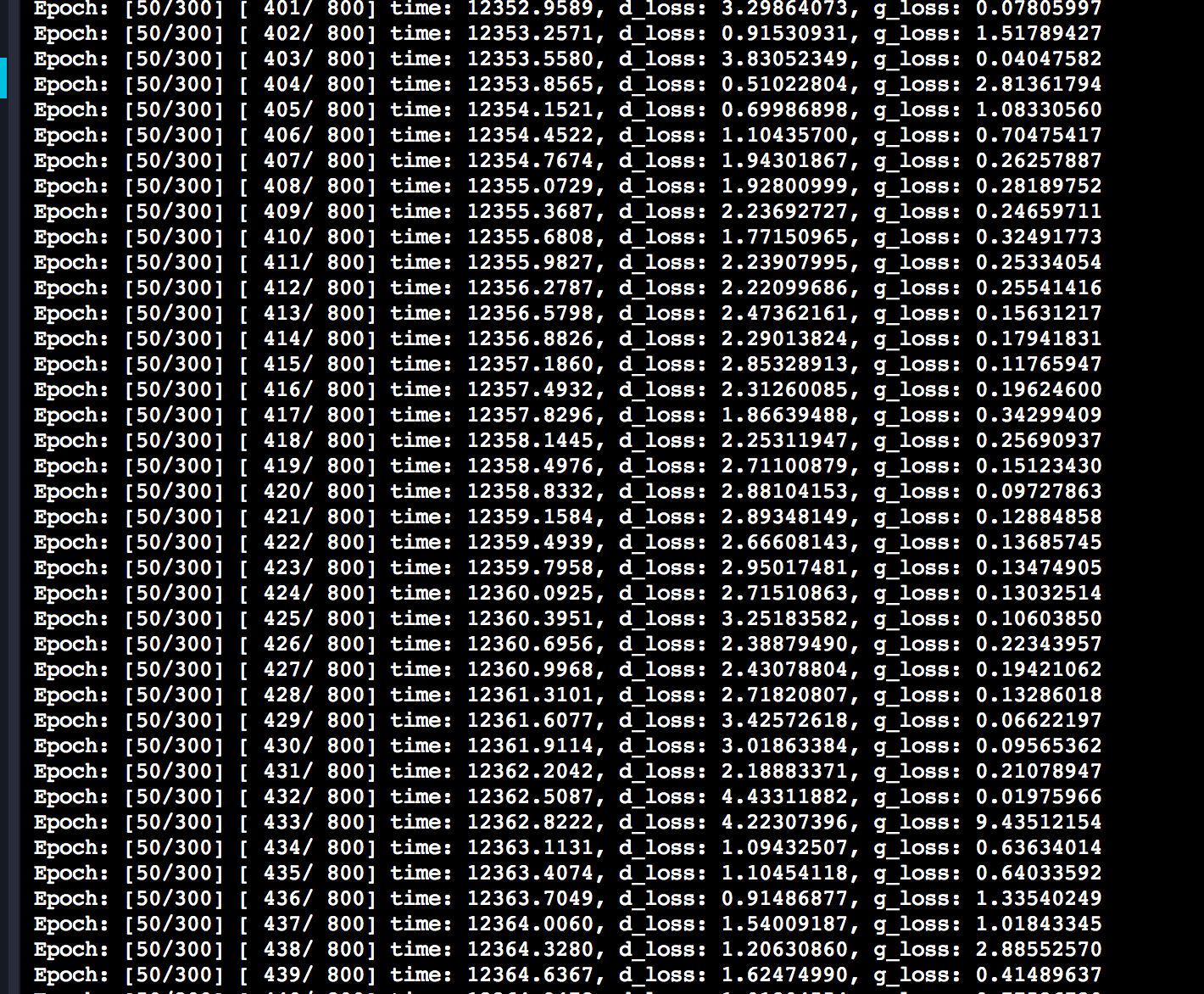epoch=1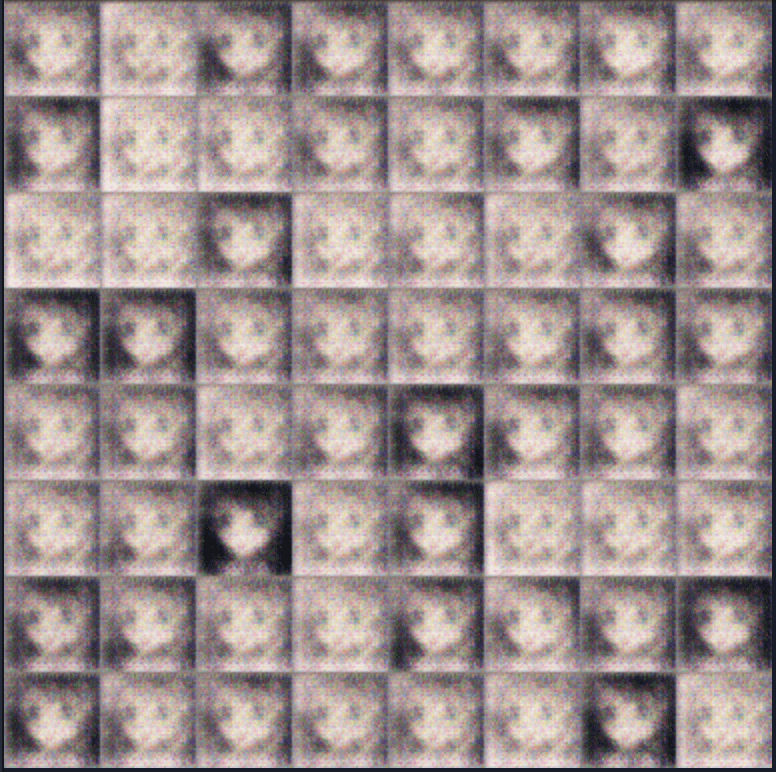epoch=30epoch=100## 总结+ 关注